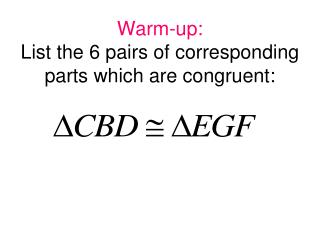Download PresentationWarm-up: List the 6 pairs of corresponding parts which are congruent:

# Warm-up: List the 6 pairs of corresponding parts which are congruent: - PowerPoint PPT PresentationDownload Presentation## Warm-up: List the 6 pairs of corresponding parts which are congruent:

- - - - - - - - - - - - - - - - - - - - - - - - - - - E N D - - - - - - - - - - - - - - - - - - - - - - - - - - -
##### Presentation Transcript

1. Triangle Congruence: SSS and SAS

2. Activity 1: Triangle Congruence • Measure and cut six pieces from the straws: two that are 2 inches, two that are 4 inches long, and two that are 5 inches long. • Cut two pieces of string that are each about 20 inches long. • Thread one piece of each size of straw onto each piece of string. Tie the ends of the string together so that the pieces of straw form a triangle. • Using the remaining pieces try to make another triangle with the same side lengths that is NOT congruent to the first.

3. E F H G Is it possible to make two triangles that have the same side lengths but are not congruent? Why or why not? SSS Congruence Conjecture If three sides of one triangle are congruent to the three sides of another triangle, then ___________. Example:

4. Warning: No AAA Postulate There is no such thing as an AAA postulate! 6 5 NOT CONGRUENT

5. Included Angle: An angle that is included between two sides of a triangle. Slides 34 - 37

6. Activity 2: Triangle Congruence • Measure and cut two pieces from the straw one that is 4 in. and one that is 5 in. • Use a protractor to help you bend a paper clip to form a 30 degree angle. • Place the pieces of straw on the sides of the angle. The straws will form two sides of the triangle. • Without changing the angle, use a piece of straw to make the third side. Use string to hold the three sides together. • Suppose you know two side lengths of a triangle and the measure of the angle between these sides. Can the length of the third side be any measure? • How does this provide a shortcut for proving triangles congruent?

7. SAS Congruence ConjectureIf two sides and the included angle of one triangle are congruent to two sides and the included angle of another triangle, then __________________. Example: A B C D

8. EX 1: Determine whether the triangles are congruent, and name the congruence shortcut you used.

9. EX 2: Determine whether the triangles are congruent, and name the congruence shortcut you used.

10. EX 3: Determine whether the triangles are congruent, and name the congruence shortcut you used.

11. EX 4: Determine whether the triangles are congruent, and name the congruence shortcut you used.

12. EX 5: Determine whether the triangles are congruent, and name the congruence shortcut you used.

13. EX 6: Show that the triangles are congruent for the given value of the variable. ∆MNO  ∆PQR, when x = 5.